and ortutorials

# laser

Laser basics

Lasers are coherent light sources based on light amplification. Light amplification is realized in a gain medium by stimulated emission. Gain medium are made of atoms with at least two energy levels ( a high and a low energy level ). Stimulated emission is achieved when an atom in high energy level absorbs a photon when emitting two photons and collapsing into its low energy level. All photons have the same wavelength corresponding to the energy gap of the transition. Stimulated emission is altered by the absorption of photons by atoms in the low energy level. Amplification occurs when stimulated emission effect is stronger than absorption. This is achieved when the atoms density is larger in the high energy level than in the low one ( population inversion ). Naturally, there are more atoms in the low energy level. Consequently, gain medium requests some exterior energy ( pump energy ) for creating population inversion.

Another phenomenom exists in a gain medium : it's the spontaneous emission. This happens when atoms in high energy level emit a photon and collapse into low energy level. Spontaneous emission is not part of the coherent light emission process and is considered as optical noise. Amplification alone is not sufficient to explain laser behaviour.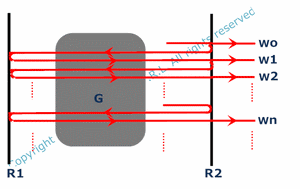The gain medium is placed in a resonant cavity. In its simplest configuration, the resonant cavity is a Fabry Perot resonator made of two mirrors. Light wave is amplified by the gain medium and reflected back and forth by the mirrors. At least, one mirror is not completely reflective. It transmits part of the inside cavity wave and acts as an output coupler. Like in a Fabry perot interferometer, output wave is the combination of inside cavity wave directly transmitted by the output coupler Wo, wave W1 transmitted after one round trip, wave W2 transmitted after 2 round trip, , wave Wn transmitted after n round trips, etc. Interferences between all these waves are constructive for resonant wavelengths of the Fabry Perot cavity, which means that emitted wavelengths ( longitudinal modes ) are modes of the Fabry Perot resonator ( for which phase shift after one round trip is 2 k p, k being an integer ). Phase shift is proportional to the optical cavity length L. In the frequency space, longitudinal modes are equally separated. Unlike a Fabry Perot interferometer, there is no incident wave : wave is generated in the gain medium and no light is emitted for wavelengths different from longitudinal modes. One condition for laser emission is that wave intensity remains the same after a round trip in the cavity. In other words, amplification by the gain medium G after one round trip compensates loss by mirrors ( expressed by mirrors reflectivity R1 and R2) : G.R1.R2 = 1.

Spectrum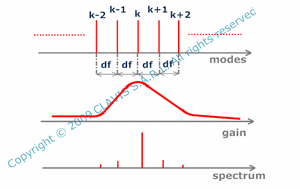k precedently defined is the longitudinal mode number. As Fabry perot interferometer, space ( df ) between adjacents modes is constant when expressed in frequencies. Most of the time, modes are calculated in wavelengths. The laser spectrum depends on the resonant cavity but also on the gain medium. The gain G is not uniform and is enabling amplification on a certain spectrum , so that finally a few longitudinal modes are emitted. It is possible also to select only one longitudinal mode in order to emit a very coherent light.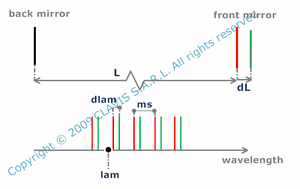In some situations, it can be interesting to calculate the shift of the longitudinal mode caused by a little cavity length change dL ( for example generated by temperature fluctuation).

In some pulsed lasers, longitudinal modes are synchronized ( mode locked lasers ). In this case, there is a relation between the spectrum width ( and consequently the modes number ) and the pulsewidth. The larger the spectrum, the shorter the pulse. The pulsewidth ( dt ) is then a function of distance between longitudinal modes expressed in the frequency space ( df ) and longitudinal modes number ( N ).

Beam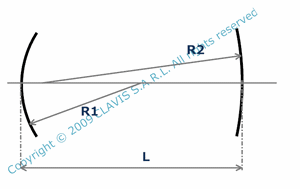The last condition for a light wave can oscillate in a laser is that its spatial intensity distribution remains the same after one round trip in the cavity. This means that the cavity is stable. It is possible to know if a cavity is stable by calculating the parameter a depending on the distance between mirrors ( L ) and the mirrors radii of curvature ( R1 and R2 ).
The cavity is stable if a is larger than 0 and smaller than 1.

Geometric optic is not sufficient to explain light emission by a laser. Electromagnetic theory with limit conditions given by spatial and phase stabilities after one round trip in the cavity impose specific emitted waves. They are called the transverse modes of the laser. They depend on mirrors shape and their relatives positions. Different models exist depending on the cavity. In the paraxial approximation, they pedict modes with different kinds of intensity distributions in the plane perpendicular to the propagation ( hermito gaussian distribution, laguerre gaussian distribution,...).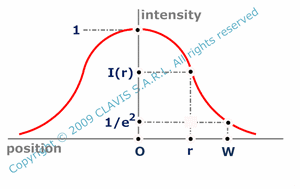In a cavity with a cylindrical symmetry, among all the modes, the fundamental mode can be approximated by a gaussian intensity distribution in the transverse plane. The fundamental mode is the less diverging mode. Consequently, emitting in the fundamental mode ( it is called later "gaussian mode" ) insures the highest power density and the highest beam spatial resolution. In a transverse plane, gaussian mode is commonly delimited by the circle containing 86,5% of the light power ( circle for which relative intensity I(r) is 1/e2 ), which radius/diameter defines radius/diameter of the beam. Beam diameter changes with the considered plane and gives an hyperboloidic beam envelope wich axis is the propagation axis.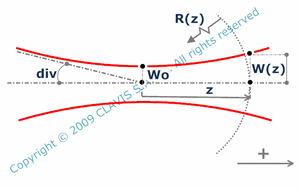Gaussian beams have some characteristic parameters. Waist is the thinnest part of the beam and Wo represents its radius. W(z) is the beam radius at a distance z from the waist. It depends on Wo and the wavelength lam. The divergence ( div ) is the angle ( with the propagation axis ) of the asymptotes of the hyperboloid and represents the beam divergence far enough from the waist position. div is a function of Wo and wavelength ( lam ) as well as zo called the Rayleigh range. At a distance smaller than zo from the waist, the beam diameter is roughly equal to the waist diameter ( beam diameter is square root of 2 times the waist diameter at zo ). At a distance much larger than zo, the beam can be approximated as issued from a point on axis at the waist position and with the divergence div. R(z) is the radius of curvature of the wavefront at a distance z from the waist. It is also a function of z, Wo and lam. The gaussian approximation of the fundamental mode propagation is suitable only for small beam divergence.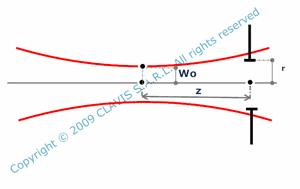Ratio ( T ) of transmitted light through a circular aperture is function of the aperture distance to the waist ( z ), the waist radius ( Wo ), the wavelength ( lam ) and the aperture radius ( r ).In some cases, for example if a gaussian beam goes through a circular aperture ( radius r ), the aperture can be placed in a range of locations on the propagation axis so far a certain given attenuation limit is not exceeded. The farest aperture position from the waist acceptable is the depth of focus ( dz ) depending on Wo, r and lam.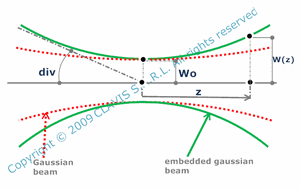In reality, laser beams are never exactly gaussian because the "gaussian theory" is an approximation, because of cavity imperfections or because the beam is a combination of a gaussian mode and other higher order modes. Supposing that the beam has a waist, its divergence is larger than the divergence of the theoretical gaussian beam with the same waist radius. A simple way to modelize the beam propagation is to consider it as an embedded gaussian which is an hyperboloidic beam which divergence is given by the divergence of the theoretical beam multiplied by the M2 factor ("M-square" factor ). M2 is necessarily larger than 1. For embedded gaussian beams, the divergence div depends on Wo, lam and M2 as well as W(z) which is also depending on z.Beams may not have a symmetry of revolution. It's the case for semiconductor lasers or when mirrors themselves don't have a symmetry of revolution. Beams have then different dimensions in the horizontal and vertical planes. They are called elliptical beams. In some cases, their fundamental mode can then be modelized by a gaussian beam with different characteristics in the horizontal and vertical planes. These are the only kind of elliptical beams considered in the calculation pages. It is possible to define the half thicknesses of the waist ( Wh and Wv ), the half thicknesses of the beam at distance z from the waist ( Wh(z) and Wv(z) ), the divergences ( divh and divv ), the Rayleigh distances ( zoh, zov ), the wavefront radii of curvature at distance z from the waist ( Rh(z) and Rv(z) ), h suffix applying for horizontal plane and v suffix for vertical plane.
Some elliptical beams may also have horizontal and vertical waists in different locations.

Beam shaping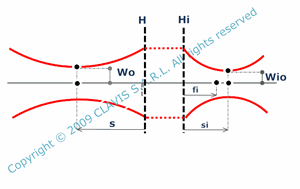Gaussian beams can be shaped by optical systems. In the gauss approximation, they remain gaussian ( assuming the optical system has a symmetry of revolution ) but with different characteristic parameters. In the general case, conjugated beam waist radius and position ( respectively given by Wio and si ) depend on incident beam waist radius and position ( respectively given by Wo and s ), wavelength ( lam ) and effective focal length of the system ( fi ).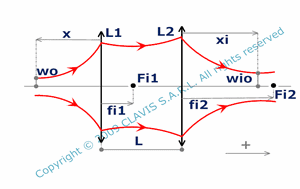When the optical system is a made of two thin lenses, conjugated beam characteristics depend on incident beam waist radius and position ( respectively Wo and x ), wavelength ( lam ), lenses focal lengths ( fi1 and fi2 ) and distance between lenses ( L ).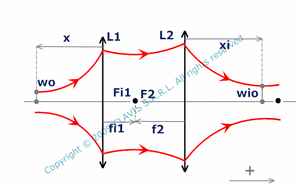When using an afocal system, they depend on incident beam waist radius and position, wavelength ( lam ), and lenses focal lengths ( fi1 and fi2 ), distance between lenses depending directly on fi1 and fi2.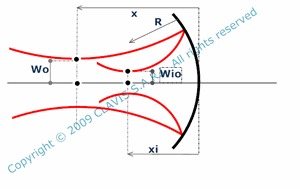Optical system can be reflective as a spherical mirror . Conjugated beam waist radius and position ( respectively Wio and xi ) are function of incicident beam waist radius and position ( respectively Wo and x ), mirror radius of curvature ( R ) and wavelength ( lam ).A cylindrical lens transforms a gaussian beam into an elliptical beam. It acts as a lens only in a transverse direction ( h ), and has no effect in the perpendicular transverse direction ( v ).Knowing the wavelength ( lam ), it's possible to calculate characteristics of an optical system transforming an incident gaussian beam with given waist radius ( Wo ) and position ( s ) into another gaussian beam with a given waist radius ( Wio ).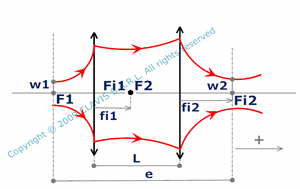In the particular case of an afocal system made of two thin lenses, if the incident beam waist is in the front focal plane of the first lens, the conjugated beam waist is in the back focal plane of the second lens. Knowing the distance between the lenses and the distance between the incident and the conjugated beam waists ( respectively L and e ), it's possible to calculate the effective focal lengths ( fi1 and fi2 ) of the two lenses adapting the beams.

In like manner, an optical system transforms embedded gaussian beams into embedded gaussian beams with different parameters. Most calculations about embedded gaussian beams shaping are very similar to gaussian beams shaping calculations evocated previously. One difference is the influence of M2 factor.

The same kind of calculations can be done also with elliptical beams. Separate calculations are made in horizontal and vertical planes. In some cases, optical systems with a symmetry of revolution are used. In other cases, the beam shaping can be made by cylindrical lenses. Whatever the optical system, one particularity of elliptical beams is that, because incident waists don't have the same width in the horizontal and the vertical planes, the conjugated beam may have horizontal and vertical waists in different locations. Furthermore, incident beam may have also horizontal and vertical waists in different locations.

Power - energy

There are two main types of lasers. Continuous lasers emit a continuous wave ( CW) and light power is constant with time.

Pulsed lasers emit pulsed waves and light power changes with time. Pulsed lasers can have different ways of functionning ( relaxed mode, Q_switch mode, mode-locked operation,etc...). Their behaviour is characterized by some useful parameters. One important parameter is the frequency ( f ) for pulses emission, which is also called the repetition rate.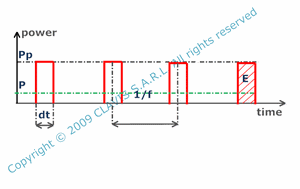Peak power ( Pp ) is the maximum instantaneous power of a pulse and can be easily calculated by assuming the power as constant during the pulse duration or pulsewidth ( dt ).

In this case, the energy per pulse ( E ) is a function of the peak power ( Pp ) and pulsewidth ( dt ).

The average power ( P ) is a function of the energy per pulse ( E ) and the repetition rate ( f ).

The duty cycle ( Dc ) is the ratio of pulsewidth ( dt ) on the period. It can also be calculated with the pulsewidth ( dt ) and the repetition rate ( f ).

References

"Cours laser" - Institut d'optique - 1986 - author : Pierre Cerez.

"Les lasers" - 1968 - author : M. Orszag.

"Cours laser et applications" - Institut Universitaire de Technologie Paul Cézanne - 2010 - author : Gilles Passedat.

"Cours résonateurs optiques et faisceaux gaussiens" - Ecole Nationale Supérieure des Télécommunications - 1988 - author : C.Debarge.

"Cours physique des lasers" - Ecole Nationale Supérieure des Télécommunications - 1988 - author : A. Migus.

"Cours sources à semiconducteurs" - Ecole Nationale Supérieure des Télécommunications - 1988 - author : J.C. Bouley.

"Understanding lasers" - 2008 - author : Jeff Hecht.

"Propagation des faisceaux gaussiens. transport des faisceaux de puissance" - Ecole d'été systèmes optiques - 2010 - author : A.Culoma.